## New Maths Curriculum: Year 2 Measurement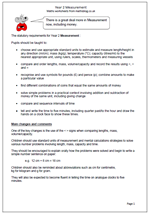The statutory requirements for Year 2 Measurement :

Pupils should be taught to:

•    choose and use appropriate standard units to estimate and measure length/height in any direction (m/cm); mass (kg/g); temperature (°C); capacity (litres/ml) to the nearest appropriate unit, using rulers, scales, thermometers and measuring vessels

•    compare and order lengths, mass, volume/capacity and record the results using >, < and =

•    recognise and use symbols for pounds (£) and pence (p); combine amounts to make a particular value

•    find different combinations of coins that equal the same amounts of money

•    solve simple problems in a practical context involving addition and subtraction of money of the same unit, including giving change

•    compare and sequence intervals of time

•    tell and write the time to five minutes, including quarter past/to the hour and draw the hands on a clock face to show these times.

One of the key changes is the use of the < > signs when comparing lengths, mass, volume/capacity.

Children should use standard units of measurement and mental calculations strategies to solve various number problems involving length, mass, capacity and time.

They should be encouraged to explain orally how the problems were solved and begin to write a simple number sentence on paper:

e.g.  12 cm + 6 cm = 18 cm

Children should also be reminded about abbreviations such as cm for centimetre,
kg for kilogram and g for gram.

They will also be expected to become fluent in telling the time on analogue clocks to five minutes.

New Maths Curriculum: Year 2 Measurement

## New Maths Curriculum: Year 1 Measurement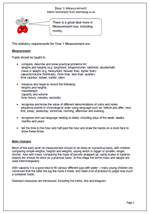Here are the statutory requirements for Measurement in Year 1. There is a great deal more in Measurement now, including money and time.

Measurement

Pupils should be taught to:

•    compare, describe and solve practical problems for:
lengths and heights (e.g. long/short, longer/shorter, tall/short, double/half)
mass or weight (e.g. heavy/light, heavier than, lighter than)
capacity/volume (full/empty, more than, less than, quarter)
time (quicker, slower, earlier, later)

•    measure and begin to record the following:
lengths and heights
mass/weight
capacity and volume
time (hours, minutes, seconds)

•    recognise and know the value of different denominations of coins and notes
sequence events in chronological order using language such as: before and after, next,
first, today, yesterday, tomorrow, morning, afternoon and evening

•    recognise and use language relating to dates, including days of the week, weeks, months and years

•    tell the time to the hour and half past the hour and draw the hands on a clock face to show these times.

Main changes

Much of the early work on measurement should to be done on a practical basis, with children comparing simple lengths, heights and weights, saying which is bigger or smaller, longer, shorter. Also with mass, comparing the mass of parcels wrapped up, using scales to balance objects etc should be done on a practical basis. At this stage the terms mass and weight are used interchangeably.

With capacity it is a good idea to fill various different jugs with water – many young children are convinced that the taller the jug the more it holds, and need a lot of practice to judge how much a container holds.

Standard measures are introduced, including the metre, litre and kilogram.

Year 1 Measurement statutory requirements

## More year 4 measurement word problems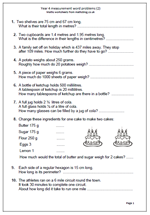Following the publication of our first set of measurement word problems for year 4 we have had several requests for some more: so here is the second page.

The questions cover a range of measurements, including converting centimetres to metres  and working with millilitres, grams and even miles. Space is given to write the answers, although children may need to make jottings or even use standard written methods to calculate some of the answers.

Most of these questions are ‘one-step’ in that only one maths operation needs to be done to find the answer, which does make them slightly easier, although children do seem to struggle with word problems generally.

Measuring word problems (2)

## Year 4 measurement word problems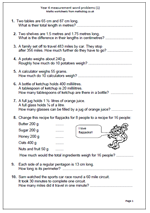Word problems can cause children quite a few problems as they have to work out what mathematical operation(s) to carry out to reach the right answers. This page is a set of word problems all to do with measurement, including time.

In order to reach the correct answers children need to be able to calculate and know about metric measurements. For example, question 1 asks:

Two tables are 65 cm and 87 cm long. What is their total length in metres.

Not only do they have to add 65 and 87 to get 152, but then convert this to 1.52 metres.

Other questions involve calculating in quarters and doubling the weight of the ingredients for making flapjacks; an early introduction to ratio.

Measuring word problems (1)

## Worksheet on problems involving measurement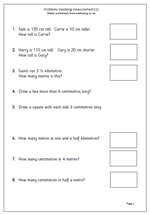By the end of year 3 children should be confident with using comparative terms such as taller, shorter, longest etc. They should know that:

1 kilometre = 1000 metres

1 metre = 100 centimetres

1 kilogram = 1000 grams

1 litre = 1000 millilitres

They should also begin to recognise simple fractions and convert between units, such as that 3 and a half kilometres is 3500 metres.

Rulers should be used to a fair degree of accuracy.

This worksheet covers some aspects of this and would be a good assessment or homework sheet.

Measurement problems

## Year 4 resources on measurement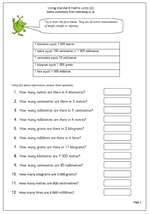We have a growing number of Year 4 resources on measurement. Of course, children today grow up learning about metric measurements and the key standard units they need to know include:

1 kilometre = 1 000 metres

1 metre = 100 centimetres

1 centimetre = 10 millimetres

1 kilogram equals 1 000 grams

1 litre = 1 000 millilitres

Our measurement worksheets reflect this and include some great ideas on practical measuring using grams, kilograms etc.

We also have a couple of pages on measuring time; these look at days and months of the year.

Go to our Year 4 measuring worksheets

## Maths worksheet: Converting metric unitsWe have a growing number of worksheets on measurement and in particular the metric system. This is about as hard as it gets for converting larger metric units to smaller ones. The larger units all include decimals and a good knowledge of multiplying by 10, 100 or 1000 is needed.

As a special treat the last question is really tricky; How many centimetres in 1.9 kilometres. I imagine that there are quite a lot of adults who would struggle with that one, but taken in logical steps it proves to be not so difficult. Find the number of metres in 1.9 kilometres, then find the number of centimetres in that amount. easy eh. It is quite interesting to look at other distances in terms of centimetres: journey home from school, length of a football pitch etc which would make for an interesting investigation.

This can be found in our Year 6 Measuring section

Year 6 Convert larger metric units to smaller (pg 2)

## Year 3 maths worksheet: Using correct units of measurement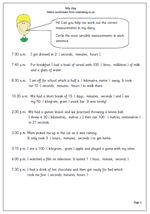Here is something slightly different from the usual maths worksheet. It is a diary of events which assesses how well children understand units of measurement and whether they can choose the most suitable unit or not.

The units include:

time: seconds, minutes, hours, days

capacity: millilitres, litres

length: centimetres, metres, kilometres

Children have to circle the most sensible of the units of measurement shown. (No Imperial measures!)

This is suitable for Year 3 children and can be found in our Year 3 Measuring section.

My day: chose suitable units of measurement

## Resource of the Week: Measuring using the metric system

Thanks to MathSphere and Mathsgogo for these two worksheets which show how much easier the metric system is than the old Imperial system. Take length for example: no 12 inches in a foot, 3 feet in a yard or 1760 yards in a mile. It’s all tens, hundreds and thousands! By Year 4 (8/9 yrs old) children should know the standard units of measurement for length, including the abbreviations.

mm (millimetres) cm (centimetres) m (metres) and km (kilometres) .They should also know that:

1 kilometre = 1 000 metres

1 metre = 100 centimetres or 1 000 millimetres

1 centimetre = 10 millimetres

These facts really do have to be learned, but it is not too difficult as they are all multiples of 10, unlike the old Imperial system!

They should also be developing their understanding of what is the best unit to use: eg what is the most suitable unit to measure the length of the garden?

It is not so easy to find real life situations where kilometres are used – certainly in athletics, cycling races etc, but unfortunately our roads are still signposted in miles – but that issue is for another whole series of blogs!

Why standard units?

Using kilometres and millimetres

## Resource of the Week: Metric worksheets for Year 4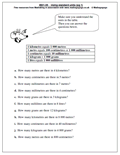We have been using metric measurement for a long time now yet many children still seem confused when measuring, whether it is distance, weight or capacity.

There are a few simple facts that children need to know, including:

1 kilometre equals 1000 metres

1 kilogram = 1000 grams

1 litre equals 1000 millilitres

Not too tricky and much easier than the old imperial unts where 1760 yards equals one mile!!

There are a number of excellent metric worksheets for Year 4, thanks to Mathsgogogo and MathSphere for allowing me to ‘borrow’ them.

Year 4 metric worksheets